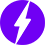# How to use load cells – Open Electronics## The sensors and electronics needed to measure the weight of objects in many areas, from electronic scales to industrial weighers.

When we talk about measuring the weight of an object, we mean the detection of the force it exerts on the detection system, whatever it is, due to the effect of earth’s gravity (force of gravity); that’s why in Physics a distinction is made between weight and mass, since the latter is the consistency of a body, while weight is the perception that mass transmits in the form of force exerted by the acceleration of gravity. It is no coincidence that mass and weight are related to the acceleration of gravity, which on average is 9.81 m / s². From this discourse it follows that measuring a weight means detecting a force, which not by chance we call force-weight. Since we are electronic, we are mainly interested in electronic weight measuring devices and among these we have pressure sensors (which are actually able to detect a force, albeit per unit area) and load cells, which are currently the sensors of choice for detecting the weight in many areas, from electronic scales to industrial weighers.

A load cell is a device that is used to measure weight and, consequently, the forces exerted, both in compression and in traction. In this article we will explain what it is and how we use this device.

## How the load cell works

When a force is applied to it in a specific way, a load cell produces an output signal that is proportional to the force itself. But how does it generate this signal? Well, the device is based on components called strain gauges, which work by exploiting the piezoresistive effect, i.e. the tendency that some electrically conductive materials have to change their resistivity when they are compressed or expanded along with certain directions. This phenomenon is similar to the piezoelectric effect that occurs in quartz and in some synthetic ceramic materials, where traction or compression produces a potential difference between two opposite faces.

A strain-gauge (strain gauge) load cell consists of an elastic element that is selectively weakened to create relatively high voltage regions; strain gauges are applied in these regions. These are connected in various configurations, although the most appreciated is the Wheatstone Bridge one, formed by four strain gauges arranged as shown in Fig. 1; in some cases, the strain gauges are two and the other two elements are common resistances. The strain gauges can also be arranged to form half-bridges and in this case, we speak of half cells.Fig.1

The Wheatstone bridge is powered between the upper and lower nodes (+/- EXCITATION points) by a continuous and stabilized excitation voltage, while the output signal is drawn between the horizontal nodes of the bridge; since the Wheatstone bridge is basically a double resistive divider from which the output potentials raised by ground are taken, the resulting voltage is the difference between them. In the diagram in Fig. 1, the output is located between A and B.

Without any load applied to the cell, all strain gauges have the same resistance and therefore there is no difference in potential between points A and B.

When the load is applied to the cell, the resistance of the voltage indicators increases, while that of the compression indicators decreases, so that the bridge becomes unbalanced and there is a potential difference proportional to the applied load, which can be measured through points A and B.

Some load cells and indicators have detection cables which in the aforementioned Fig. 1 are called + and – SENSE and which allow the indicator to measure and adjust the excitation voltage applied to the cell; this is particularly important when, for placement, the cells must be connected with long cables.

The load cells can be used individually or in combinations depending on the geometry of the object to be weighed.

Fig. 2 illustrates a typical metal strain gauge, which consists of a metal foil engraved on parallel grid lines, which form a circuit delimited by the exit pads; the foil is bonded to an insulating support material which, in turn, is constrained to the surface of the load cell, as it appears in Fig. 2.Fig. 2

In strain gauge load cells, one end of a load cell is typically attached to a rigid structure, while the other is applied to a load receiving device through which the load or force to be measured is applied; in this case it is a double point cell, which we can assimilate to a cantilever beam, stuck at one end and stressed to the other. This type of load cell is of the most common for its simplicity and low cost. The structure is often binocular, i.e. a beam with two holes. The strain gauges are mounted on the top and bottom to measure tensile and compressive forces. Since strain gauges can be damaged, they are typically covered and repaired by a rubber bellow. The beam itself is often made of nickel-plated steel alloy; for light loads (up to 230 grams), the beam is in copper-beryllium, while for higher loads it is in stainless steel. In these models, strain gages are typically protected by a urethane coating.

In Fig. 3 we see a beam load cell which shows the four strain gauges available, signed T and C and arranged, crossed, on the two faces of the metal structure weakened in the central area to facilitate deformation. With the arrangement shown, two strain gauges measure the tensile stress and two detect the compressive stress, exactly as occurs when a load (weight) is applied to the load cell. Being four, the strain gauges are connected together to form a Wheatstone bridge as illustrated in the previously seen Fig. 1, however in this case their arrangement is such as to make them collaborate, otherwise the change in resistance in one would contrast that in the other; collaborating means that the two T’s are opposite each other in the bridge, so as to obtain an imbalance when applying force.

A stabilized excitation voltage is applied to opposite corners of the Wheatstone bridge and a signal is measured on the others, points A and B.

In addition to the type proposed in Fig. 3, which works in shearing, there is the one shown in Fig. 4, which works in traction (tension cell) and is used for example in the weighing of tanks and hoppers, where the load cells are suspended from an aerial structure and the object to be weighed is hung from the lower side. This structure is practically an S and is widely used in the conversion of mechanical to electromechanical scales. In this situation, the cell is used to detect the voltage of the rod that connects the lever system to the spoke.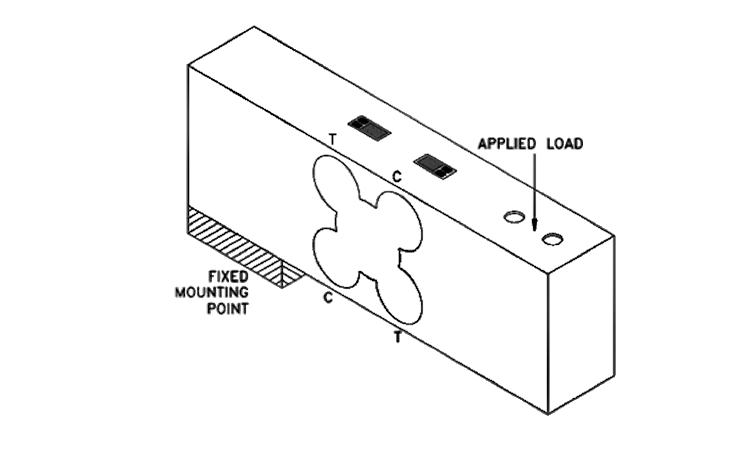Fig.3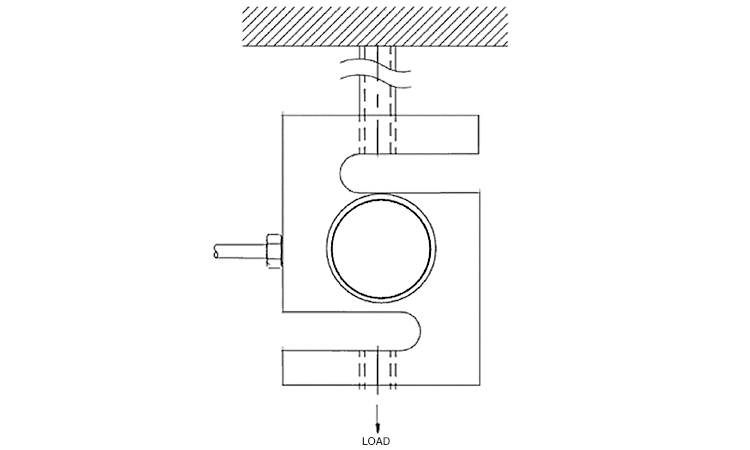Fig.4

The typical capacities for this type of cell range from 45 kg to 22 tons, while the beam ones range from about 500 g to tens of kg.

Then there are the single point load cells (Fig. 5) which are widely used in platform scales; they detect the weight regardless of where the load is applied to the upper platform. The capacities typically range from 1 kg to 2 tons and can accommodate platforms with a surface area of ​​several hundred cm2.Fig. 5

It is possible to classify the load cells according to the direction of application of the force, therefore we have the types of tension, compression, alternation and flexion (Fig. 6): the first is typically S-shaped, while the second is a button or barrel; the third works both in traction and compression and the fourth is beam.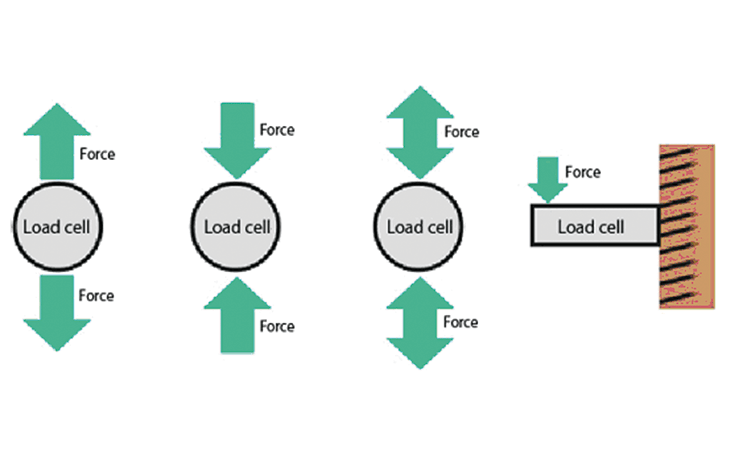Fig. 6

There is also a classification for measurement accuracy: Ultra-precise, Precise, Standard.

The shape of the spring material depends on the characteristics of the load cell.

Another classification can be made for airtightness, as set out below.

Sealed: this type encloses the areas of the strain gages inside a case and protects them from the environment. In general, the interior of the case is full of inert gas, which makes these devices suitable for use in unfavorable environmental conditions, such as outside, in the presence of dust or high humidity. A diaphragm or bellows is used so as not to affect the bending of the spring material.

Open: in it the strain gauges are not protected or at most are repaired by soft resin or rubber, which protects against environmental agents. It is suitable for operating in environments where the temperature is not too high and there is no excessive humidity or dust.

Explosion-Proof: thanks to its structure, this type of cell is fireproof

In addition to their composition, stress and seal, the load cells can be classified according to their shape (Fig. 7); we therefore have Beam Type (beam), Can Type (barrel), S Type (S) and Washer Type (washer or button)).

## Single-Point & Multi-Point

The load cells can also be distinguished in single-point and multi-point: the single-point or single-point type is used when it is necessary to measure using a single load cell, while the multi-point assumes the use of multiple load. With single-point cells, the weight is applied to the load cell since the corner errors are already corrected.

With the multi-point, three or four load cells are generally placed in various points of the structure and their signals are suitably added to correct any angle errors of the applied force.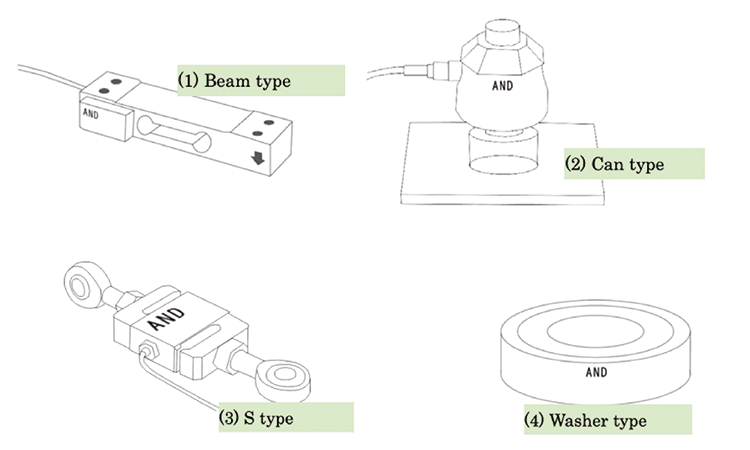Fig. 7

To read the force or weight through the load cells, it is first necessary to evaluate the most suitable configuration, then traction cells to detect hanging weights or compression to weigh objects that weigh on the system; moreover it is necessary to see what kind of precision is needed and from which direction the force comes.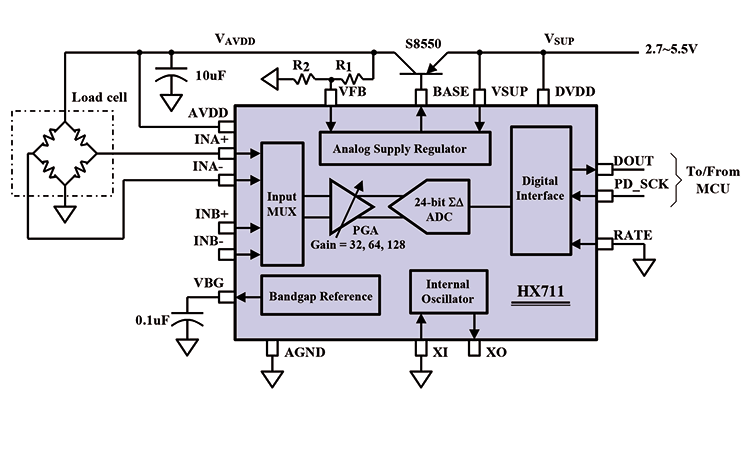Cells with only two strain gauges allow to detect of a certain type of forces and require the bridge connection with two fixed resistors, while those with four strain gauges, arranged crosswise, form a complete Wheatstone bridge from them.

The signals provided by the bridge, in both cases, are very small; especially those of the cells with only two strain gauges, since those of the cells with four strain gauges, by connecting the elements in an appropriate manner, provide differential signals, which therefore have greater amplitudes.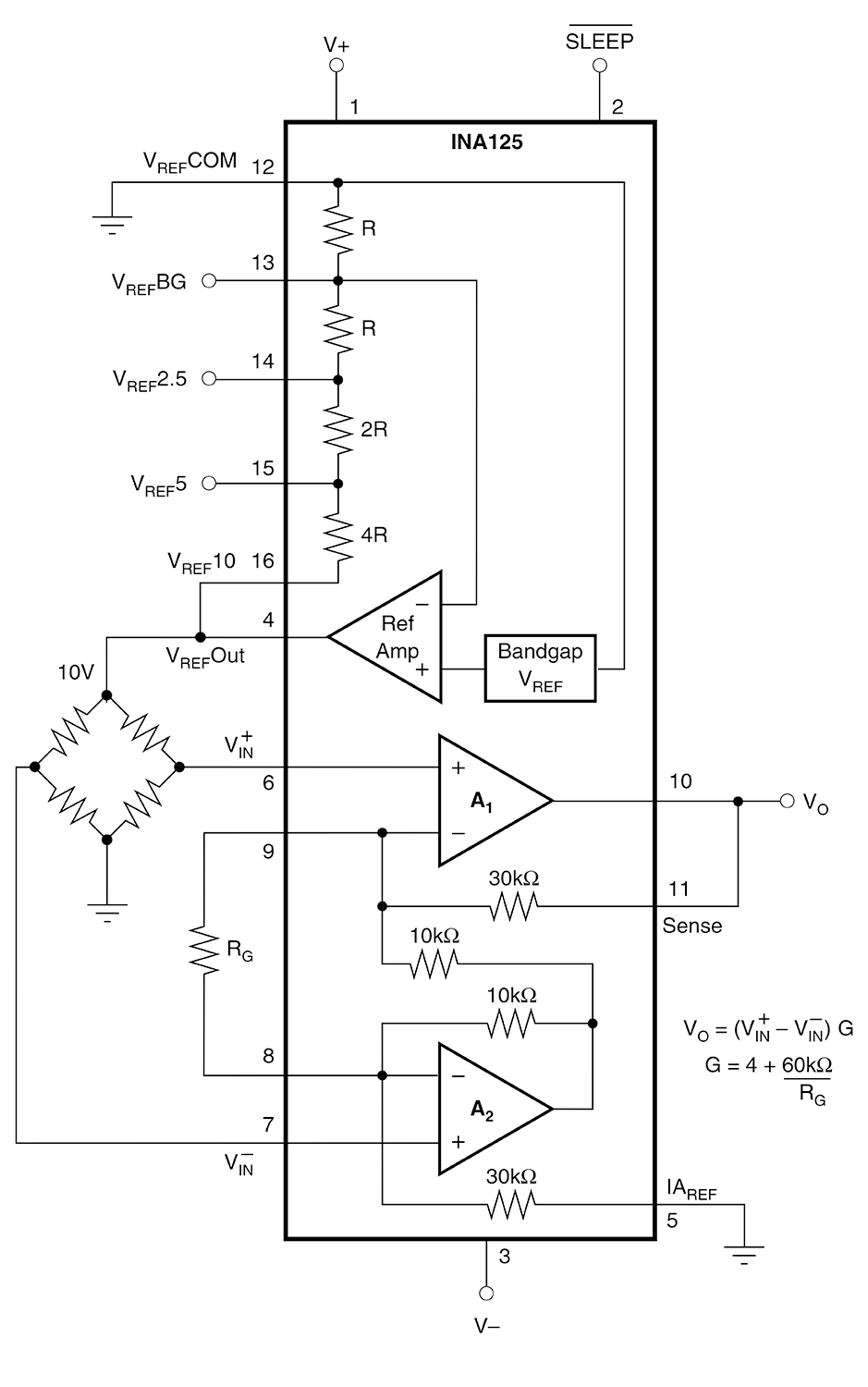In any case, it is unthinkable to read the signal directly with the ADC of a microcontroller or with Arduino, but conditioning should be carried out. This term means amplification just enough to raise the level to values ​​that can withstand the disturbances that may enter the connections, and to reduce any radioelectric interference.

There are specific integrated circuits or in any case suitable for the signal conditioning of strain gage load cells: for example, INA125 or HX711; the characteristics of both are shown in the boxes dedicated to them on these pages.Of course, the Arduino public finds load cells interesting and that’s why we thought of creating some simple applications to propose; it should be immediately specified that to read with Arduino the signal offered by the load cells, it is necessary to interpose a conditioning circuit and for this purpose the two integrated ones just described are suitable. In the applications described, we will use a breakout that mounts the HX711 (code HX711BOARD) and the 500g load cells code LOADCELL500 which we will connect to Arduino as shown in Fig. 11.Fig. 11

As you can see, the cell has four wires and connects as shown. From the output of the breakout board, which draws power from the Arduino 5V (and in turn feeds the load cell), the digital signal containing the information on the weight force is taken. More exactly, the breakout board provides data strings on a two-wire I²C type bus through the DT (data) line synchronized with the clock that Arduino generates on the SCK line. Listing 1 offers the code necessary to acquire the readings taken through the load cell and the HX711.

Listing 1

```#include “HX711.h”
#define calibration_factor -9580.00 //This value is obtained using
//the SparkFun_HX711_Calibration sketch
#define DOUT 4
#define CLK 5
HX711 scale(DOUT, CLK);
void setup() {
Serial.begin(9600);
Serial.println(“HX711 scale demo”);
scale.set_scale(calibration_factor);
scale.tare(); //Assuming there is no weight on the scale
//at start up, reset the scale to 0
}
void loop() {
float libbre = scale.get_units();
float kg = (libbre/2.2046);
Serial.print(kg, 1); //scale.get_units() returns a float
Serial.print(“ kg”); //You can change this to kg but you’ll need
//to refactor the calibration_factor
Serial.println();
}```

Let’s now move on to a second application example based on a different configuration, i.e. 2 half-cells loaded with a capacity of 50kg each (code LC50KG), for a total capacity equal to 100kg. Electrically, these half cells make up each half of the Wheatstone bridge and are connected via the usual breakout board based on the HX711 as shown in Fig. 12.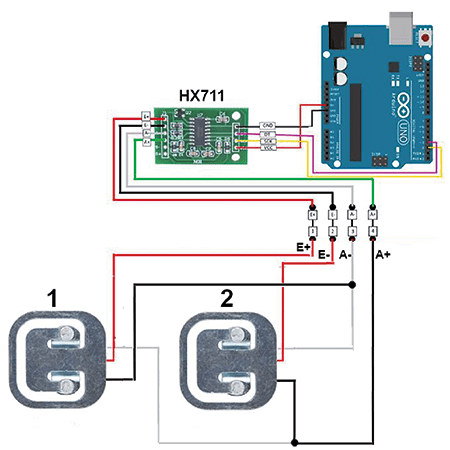Fig. 12

These load cells have the following characteristics:

output signal = 1 mV (± 0.1);

non-linearity = 0.05 (% on full-scale);

hysteresis = 0.05 (% on full scale);

repeatability = 0.05 (% on full scale);

creep after 1 minute = 0.1 (% on full scale);

effect of temperature on the outlet = 10 ° C (0.1% on the full scale);

effect of temperature on zero = 10 ° C (0.3% on full scale);

input resistance = 1 kohm (± 10);

output resistance = 1 kohm (± 10);

insulation resistance> 2,000 Mohm

operating temperature = from -10 ° C to + 40 ° C;

bridge excitation voltage = 10 V;

dimensions (mm): 34x34x8.

For Arduino connections, remember that the red wire is the positive (VCC) black the negative (GND) and the white the output (OUT) to be connected as shown.

The Arduino code to perform the weight measurement with initial tare determination can be found in Listing 2 and includes the HX711 library.

Listing 2

```#include “HX711.h”
#define calibration_factor -9580.00 //Calibration factor, obtained
//Using the sketch SparkFun_HX711_Calibration
#define DOUT 4 //Digital pin DT
#define CLK 5 //Digital pin SCK
HX711 scale(DOUT, CLK);
void setup() {
Serial.begin(9600);
Serial.println(“Rilevazione Peso con Tara iniziale”);
scale.set_scale(calibration_factor);
scale.tare(); //Make sure there is no weight at the start.
delay(500);
Serial.println(“Tara in corso... non mettere nessun peso!”);
delay(1000);
Serial.println(“Sistema pronto.”);
delay(300);
}
void loop() {
Serial.print(“Peso: “);
float Pounds = scale.get_units();
float kg = (libbre/2.2046); //Conversion weight Pounds in Kg
Serial.print(kg, 1);
Serial.print(“ kg”);
Serial.println();
}```

## Circuit with four half cellsFig. 13

From the previous configuration, we have obtained a further system, however, consisting of 4 half-load cells of the same type, which could be used to detect the weight of a crate resting on the half-cells, each arranged on a corner of a structure such as that proposed in Fig. 13. Being the load cells (half cells) each capable of weighing 50 kg, we can reach a maximum of 200 kg in a perfectly balanced way, since we have the elements on the four corners. The circuit to be created with Arduino is described in the wiring diagram proposed in Fig. 14; the red cable is “picked up” from each cell and connected to the pins of the HX711 board. This Wheatstone bridge configuration allows having a balanced system in which the weight is measured in a balanced way. The code for Arduino relating to the latter application example is proposed in Listing 3.Fig. 14

Listing 3

```#include “HX711.h”
#define DOUT 4
#define CLK 5
const int buttonPin = 2;
int buttonState = 0;
HX711 scale(DOUT, CLK);
float calibration_factor = -9640.00; //-7050 worked for my 440lb max scale setup
void setup() {
Serial.begin(9600);
Serial.println(“HX711 calibration sketch”);
Serial.println(“After readings begin, place known weight on scale”);
Serial.println(“Press + or a to increase calibration factor”);
Serial.println(“Press - or z to decrease calibration factor”);
scale.set_scale();
scale.tare(); //Reset the scale to 0
Serial.print(“Zero factor: “); //This can be used to remove the need to tare the scale. Useful in permanent scale projects.
Serial.println(zero_factor);
pinMode(buttonPin, INPUT);
}
void loop() {
if (buttonState == HIGH) {
scale.set_scale();
scale.tare(); //Reset the scale to 0
Serial.print(“Zero factor: “); //This can be used to remove the need to tare the scale.
Serial.println(zero_factor);
pinMode(buttonPin, INPUT);
}
scale.set_scale(calibration_factor); //Adjust to this calibration factor
float libbre = scale.get_units();
float kg = (libbre/2.2046);
Serial.print(kg, 1); //scale.get_units() returns a float
Serial.print(” kg”);
Serial.print(” calibration_factor: “);
Serial.print(calibration_factor);
Serial.println();
if(Serial.available())
{
if(temp == ‘+’ || temp == ‘a’)
calibration_factor += 10;
else if(temp == ‘-‘ || temp == ‘z’)
calibration_factor -= 10;
}
}```

## From openstore

Arduino UNO R3

Weight shield

Electronic board for Load Cell – HX711

We will be happy to hear your thoughts